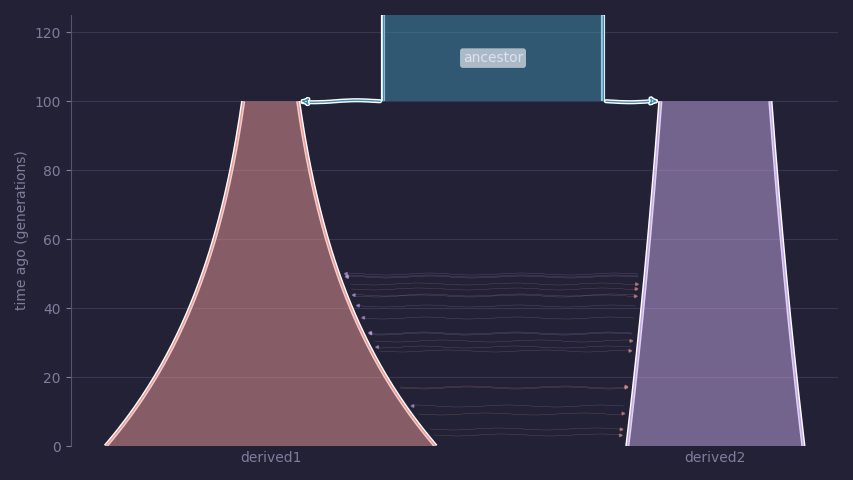# Demes: a standard format for demographic models

This post discusses a recent paper resulting from a wonderful collaboration where we attempt to define a standard way to describe a specific family of models that are widely used in population genetics.

## Background

Population genetics research heavily depends on software for two tasks:

• Making inferences from data.
• Simulating data.

These two tasks are often tightly coupled — we will simulate data to test our inference methods, etc..

The field’s software ecosystem is highly fragmented. Conservatively, dozens of tools exists for both tasks. To some extent, this is reasonable. The problems are hard and academic research tends to slowly grind away on them bit by bit over the years.

One challenge of this fragmentation is that most of the tools cannot interoperate. Consider the following work flow:

• Parameters of a multi-population model are inferred using moments or dadi.
• These parameters are used to simulate data using one or more of:
• Backwards in time using ms
• Backwards in time using msprime
• Forwards in time using SLiM
• Forwards in time using fwdpy11
• The simulation output is used to make statements about how well the fitted models match up with the data.

All of the tools just mentioned are used by research currently in progress. All of them have different ways of saying, “we want to do something with a model of an ancestral population that split into two populations in the recent past”. We have a mix of command line tools, tools with Python APIs, tools requiring input files in a bespoke format, etc..

This has been the situation for the past 20+ years. The lack of interoperability has real consequences. A lot of time can be lost (wasted) dealing with the complexities of changing the model description to use with different tools. The process is error-prone and has led to errors.

`demes` is an effort to do better.

## Example model

Consider the following scenario:

• An ancestral population of 1,000 individuals splits into two populations 100 generations ago.
• The two derived populations have different initial sizes and grow exponentially to different final sizes.
• Symmetric migration occurs between the two derived populations over the last 50 generations.

Instead of learning the `ms` command line or the `msprime` demographic events API, let’s just write our model down in `YAML`, a standard format for data interchange:

``````description: population split with bottlenecks and growth
time_units: generations
demes:
- name: ancestor
epochs:
- start_size: 1000
end_time: 100
- name: derived1
ancestors: [ancestor]
epochs:
- start_size: 250
end_size: 1500
- name: derived2
ancestors: [ancestor]
epochs:
- start_size: 500
end_size: 800
migrations:
- demes: [derived1, derived2]
rate: 1e-5
start_time: 50``````

The advantages of using `YAML` include:

• It is independent of the API of any specific simulation or inference software. Thus, one input file can be used with many tools.
• Every modern programming language can easily parse `YAML`.

The following graphic illustrates the model. Time on the y axis starts 0 generations ago, indicating the present day.The code to generate this graphic is:

``````import matplotlib.pyplot as plt

import demes
import demesdraw

tubes = demesdraw.tubes(graph)

plt.savefig("demes_post_model.png")``````

## Specification and software ecosystem

The complete specification of the `demes` format is here. The paper discusses the reasons behind several of the decisions in the spec. The paper also lists what tools currently use `demes` as input and/or output formats. Graham Gower has written an excellent tutorial.

`demes` has good language support:

• python
• demesdraw may be the shining star of all the tools so far! We can finally visualize our models with a line or three of Python.
• C
• julia
• rust

## Integration with fwdpy11

fwdpy11 is developed by our group. We can define demographic models using `demes` quite easily:

``````# Read the model in from the YAML.
# The 1 tells fwdpy11 to generate
# a model where the ancestral population is
# evolved for N generations, where N is its initial size
model = fwdpy11.discrete_demography.from_demes("demes_post_model.yaml", 1)``````

### How the sausage used to be made

Prior to `demes`, the following Python code was needed to define this same model in `fwdpy11`:

``````model=fwdpy11.DiscreteDemography(
mass_migrations=[],
set_growth_rates=[
fwdpy11.SetExponentialGrowth(when=1000, deme=1, G=1.0180790778133073),
fwdpy11.SetExponentialGrowth(when=1000, deme=2, G=1.0047110987876184),
],
set_deme_sizes=[
fwdpy11.SetDemeSize(when=1000, deme=0, new_size=0, resets_growth_rate=True),
fwdpy11.SetDemeSize(
when=1000, deme=1, new_size=250, resets_growth_rate=True
),
fwdpy11.SetDemeSize(
when=1000, deme=2, new_size=500, resets_growth_rate=True
),
],
set_selfing_rates=[
fwdpy11.SetSelfingRate(when=0, deme=0, S=0),
fwdpy11.SetSelfingRate(when=1000, deme=1, S=0),
fwdpy11.SetSelfingRate(when=1000, deme=2, S=0),
],
migmatrix=fwdpy11.MigrationMatrix(
migmatrix=array([[1.0, 0.0, 0.0], [0.0, 0.0, 0.0], [0.0, 0.0, 0.0]]),
scaled=False,
),
set_migration_rates=[
fwdpy11.SetMigrationRates(
when=1000, deme=0, migrates=array([0.0, 0.0, 0.0])
),
fwdpy11.SetMigrationRates(
when=1000, deme=1, migrates=array([1.0, 0.0, 0.0])
),
fwdpy11.SetMigrationRates(
when=1000, deme=2, migrates=array([1.0, 0.0, 0.0])
),
fwdpy11.SetMigrationRates(
when=1001, deme=1, migrates=array([0.0, 1.0, 0.0])
),
fwdpy11.SetMigrationRates(
when=1001, deme=2, migrates=array([0.0, 0.0, 1.0])
),
fwdpy11.SetMigrationRates(
when=1050, deme=1, migrates=array([0.0000e00, 9.9999e-01, 1.0000e-05])
),
fwdpy11.SetMigrationRates(
when=1050, deme=2, migrates=array([0.0000e00, 1.0000e-05, 9.9999e-01])
),
],
)``````

It should be obvious which method to use…

## Future directions

`fwdpy11` is moving towards a situation where `demes` graphs are the core object for evolving models of discrete populations. As of version `0.19.0`, the rust implementation of `demes` is compiled into the core library. (That is a neat story on its own, as the back-end is now a C++ dynamic library that is partially written in rust.)

The types found in the very long code listing above will be deprecated in favor of directly importing either `YAML` files or `demes.Graph` objects from Python. I am guesstimating that the changes will result in a few thousand lines of C++ and Python being deleted.

## Code

Here is the code for the above examples.